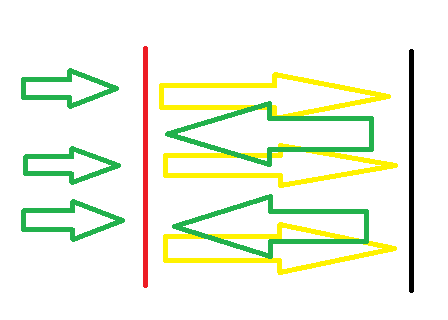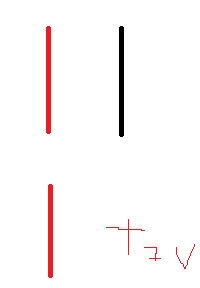# Parallel plates potential

Tryhard314
Summary:: if Plate A had a potential of 9V, This means as We approach a unit charge from +Infinity to A we have to do this precise amount of work
Now we remove plate A, And replace it with plate B that has a potential of -9V Again that means to go from +Infinity To B we actually gain energy, or in other words to go from B to +Infinity we have to do this amount of work.
Now What is the potential of A if plate B is nearby (at a distance D).

if Plate A had a potential of 9V, This means as We approach a unit charge from +Infinity to A we have to do this precise amount of work

Now we remove plate A, And replace it with plate B that has a potential of -9V Again that means to go from +Infinity To B we actually gain energy, or in other words to go from B to +Infinity we have to do this amount of work.
Now What is the potential of A if plate B is nearby (at a distance D).

(Minute 14)
Like in this video of professor Walter Lewin he explain pretty intuitively That since the potential of A is the work we have to do to bring a unit charge To A, and B is attracting this unit charge, The potential of A goes down.In my opinion this is true but only on the left side of the image, Since the attraction of the negative plates opposes the repulsion of the positive plate,But inside the separation if you want to approach the positive plate you need to overcome the repulsion of the positive plate And the attraction of the Negative plate (if you came from the right then entered the separation),

1 ) doesn't this you have to put in more work in order to approach A?

2 ) inside the separation between the plates do we have the original 18v potential difference or a lower one because outside the separation forces counter each other but inside it they add so it's difficult to understand why the potential gets lower(don't use conservation of energy please).

3 )if you approach A from the right side of + infinity it should be equal to approaching it from the left side since efield are conservative but that doesn't help me understand why.
But it does awnser partially the last question since it tells that the energy you gain approaching the negative plate helps push the positive charge inside the separation and the total network should be the same as if you approached from the left (i understood why it should be the same not WHY IT IS the same)

I numbered the questions so you can follow easily , Thanks for your time! (I thought i understood athis topic when I first studied it but when revising I got really really confused if you clarify this for me I would be grateful)

Last edited by a moderator:

willem2
While the field between the plates that are close together is twice as strong as the field of a single plate, the distance between the plates is much smaller than the distance from one plate to infinity.
Energy is force times distance.
If you bring two isolated plates closer together, the electric field doesn't change much, but the distance between the plates gets smaller, and therefore the potential difference also becomes smaller.

••Tryhard314 and PeroK
Tryhard314
While the field between the plates that are close together is twice as strong as the field of a single plate, the distance between the plates is much smaller than the distance from one plate to infinity.
Energy is force times distance.
If you bring two isolated plates closer together, the electric field doesn't change much, but the distance between the plates gets smaller, and therefore the potential difference also becomes smaller.

If i understood what you saidif we were to remove the negative plate we would still experience a positive potential at it's last position due to the positive plate A, so we need to increase the distance so much that the potential Due to plate A is near zero, Thanks very clear awnser!